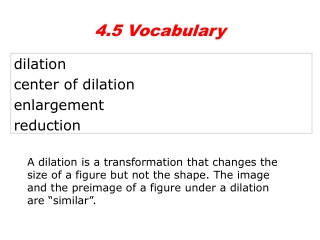DownloadDownload Presentationdilation center of dilation enlargement reduction

# dilation center of dilation enlargement reduction

Download Presentation## dilation center of dilation enlargement reduction

- - - - - - - - - - - - - - - - - - - - - - - - - - - E N D - - - - - - - - - - - - - - - - - - - - - - - - - - -
##### Presentation Transcript

1. 4.5 Vocabulary dilation center of dilation enlargement reduction A dilation is a transformation that changes the size of a figure but not the shape. The image and the preimage of a figure under a dilation are “similar”.

2. Example 1: Identifying Dilations Tell whether each transformation appears to be a dilation. Explain. A. B. No; the figures are not similar. Yes; the figures are similar and the image is not turned or flipped.

3. A dilation enlarges or reduces all dimensions proportionally. A dilation with a scale factor greater than 1 is an enlargement, or expansion. A dilation with a scale factor greater than 0 but less than 1 is a reduction, or contraction.

4. Example 2A: Drawing Dilations Copy the figure and the center of dilation P. Draw the image of ∆WXYZ under a dilation with a scale factor of 2. Step 1 Draw a line through P and each vertex. Step 2 On each line, mark twice the distance from P to the vertex. W’ X’ Step 3 Connect the vertices of the image. Y’ Z’

5. Check It Out! Example 2B Copy the figure and the center of dilation. Draw the dilation of RSTU using center Q and a scale factor of 3. Step 1 Draw a line through Q and each vertex. R’ S’ Step 2 On each line, mark twice the distance from Q to the vertex. Step 3 Connect the vertices of the image. T’ U’

6. Helpful Hint For a dilation with scale factor k, if k > 0, the figure is not turned or flipped. If k < 0, the figure is rotated by 180°.

7. If the scale factor of a dilation is negative, the preimage is rotated by 180°. For k > 0, a dilation with a scale factor of –k is equivalent to the composition of a dilation with a scale factor of k that is rotated 180° about the center of dilation. Note: When you multiply by a –k the rotation is taken care of. You do not need to rotate the points.

8. The dilation of (x, y) is Example 3A: Drawing Dilations in the Coordinate Plane Draw the image of the triangle with vertices P(–4, 4), Q(–2, –2), and R(4, 0) under a dilation with a scale factor of centered at the origin.

9. Q’ R’ P’ Example 3A Continued Graph the preimage and image. P R Q

10. The dilation of (x, y) is Check It Out! Example 3B Draw the image of the triangle with vertices R(0, 0), S(4, 0), T(2, -2), and U(–2, –2) under a dilation centered at the origin with a scale factor of .

11. T’ U’ S’ R’ S R T U Check It Out! Example 3B Continued Graph the preimage and image.

12. 2. Copy ∆RST and the center of dilation. Draw the image of ∆RST under a dilation with a scale of . Lesson Quiz: Part I 1. Tell whether the transformation appears to be a dilation. yes

13. Lesson Quiz: Part II 3. A rectangle on a transparency has length 6cm and width 4 cm. On the transparency 1 cm represents 12 cm on the projection. Find the perimeter of the rectangle in the projection. 240 cm 4. Draw the image of the triangle with vertices E(2, 1), F(1, 2), and G(–2, 2) under a dilation with a scale factor of –2 centered at the origin.

14. HONORS: Dilation Point Not the Origin… How would you perform a dilation about a point that is not the origin? Same as rotating about a non-origin point! • STEPS: • Transform the points to be dilated to a new coordinate system centered at the dilation point by subtracting the dilation point coordinates from the coordinates of the points to be dilated. • Dilate the points in the new coordinate system with the origin at the point of dilation. • Transform the dilated coordinates back to the original origin by adding the dilation point coordinates to the coordinates of the dilated points.